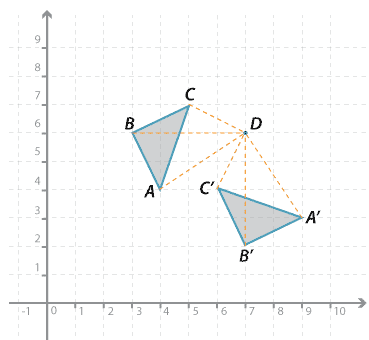## Content description

Describe translations, reflections in an axis, and rotations of multiples of 90 ° on the Cartesian plane using coordinates. Identify line and rotational symmetries (ACMMG181)

Source: Australian Curriculum, Assessment and Reporting Authority (ACARA)

There is a separate resource for line and rotational symmetry### Rotations of multiples of 90° about a pointDetailed description

In the figure above, $$\triangle ABC$$ is rotated 90 ° in an clockwise direction about point $$D$$ to the image $$\triangle A^\prime B^\prime C^\prime$$.

The point $$A$$ in the diagram above is rotated 90 ° in a clockwise direction about point $$D$$. Note that $$AD = A^\prime D$$ and $$\angle AD A^\prime = 90 ^\circ$$.

Using coordinates we can describe the rotation of the vertices:

$$A(4, 4) \rightarrow A^\prime (9, 3); B(3, 6) \rightarrow B^\prime (7, 2); C(5, 7) \rightarrow C^\prime (6, 4)$$.